# Decimal Chart PrintableUpdated on Jan 31, 2022By Printablee Team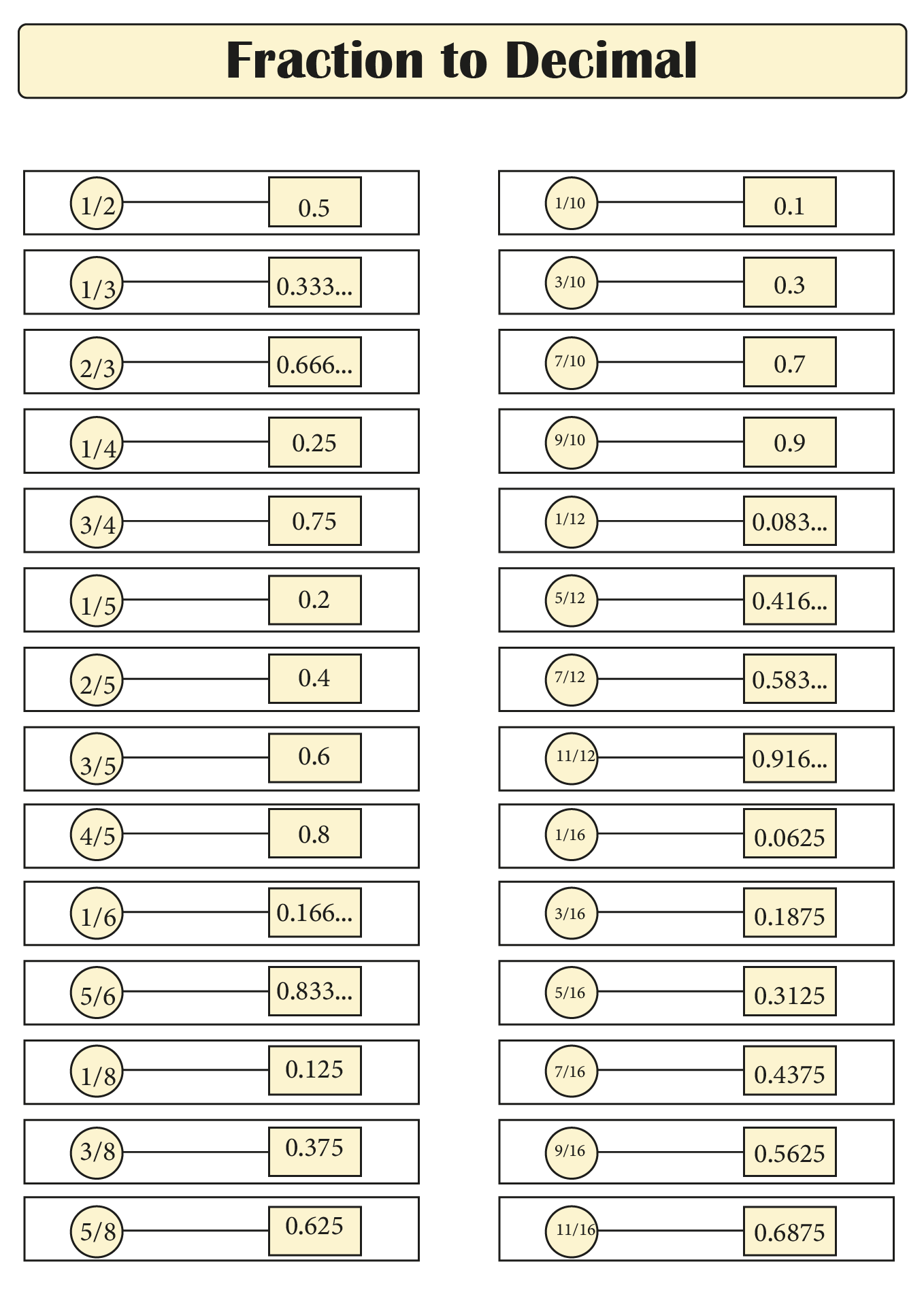## What is The Decimal Number System?

The decimal number system is a positional notation system for numbers to the ten digit. Therefore, the decimal number system is also known as the base 10 number system. That's because it uses a ten-digit system to represent all number values.

Actually, the decimal system is related to the number system in the era of Ancient India. It was in this era that the decimal system was first used. In the 5th century, an Indian mathematician, Brahmagupta, introduced the concept of zero to make arithmetic operations easier and more efficient.

After that, the decimal system was introduced to Arabic through education and trade. Then, the number system spread to Europe. In medieval times, the decimal system became a popular number system.

People used decimal fractions as a number and decimal points in the 17th and 18th centuries. That's because of the development of the decimal system created by European mathematicians, John Napier and Simon Stevin.

In general, the decimal number system has the following characteristics.

• The ten digits (0-9) are used to represent all numeric values so those numbers are easier to use and understand.
• The number system also has positional notation. So, each digit of a decimal number represents 10 digits. It helps you to do number calculations more easily because you can convert numbers into simple forms that are easy to calculate.
• Decimal numbers have a decimal point which is used to separate numbers from their fractions.
• Decimal numbers also have a place value. It is the positional value of a digit in a decimal number that can help you determine a numeric value. An example is the number 3 in the 350. The number 3 in this number has a numerical value in hundreds (300).
• Addition identity is also characteristic of decimal numbers. The zero in a decimal number is the addition identity. So, adding the number 0 will not change the value of the decimal number.

You can get a decimal number if you break the number into smaller parts. So, it can be concluded that decimal numbers are another form of fractions. Decimal numbers are obtained by placing a comma (decimal point) to the right.

## Why are Decimal Systems Often Used in Mathematics?

The decimal system is the most widely used number system in mathematics because it is based on the ability of the human finger to count. Yes, the system was created by adjusting the human fingers. This makes the decimal system convenient and intuitive to use in mathematical calculations. So, that's what makes the decimal system used the most in math.

Because of its convenience, the decimal system is used in various fields of life. It also makes the decimal system the standard mathematical and computational concept. No wonder we often use the concept of decimal numbers in everyday life.

## What are The Implementation of Decimal Systems in Real Life?

There are many implementations of the decimal system in life such as measuring oil volume and height. Because the decimal system is used in everyday life, the concept of the decimal system is an important material taught in schools. To help you understand the implementation of the decimal system, here we provide an explanation.

• Medical: The decimal system is often implemented for medical needs. An example is to measure temperature.
• Counting Money: You also often use the concept of decimals in financial calculations.
• Weight: If you calculate the weight, there is a decimal number that you will find on the scale.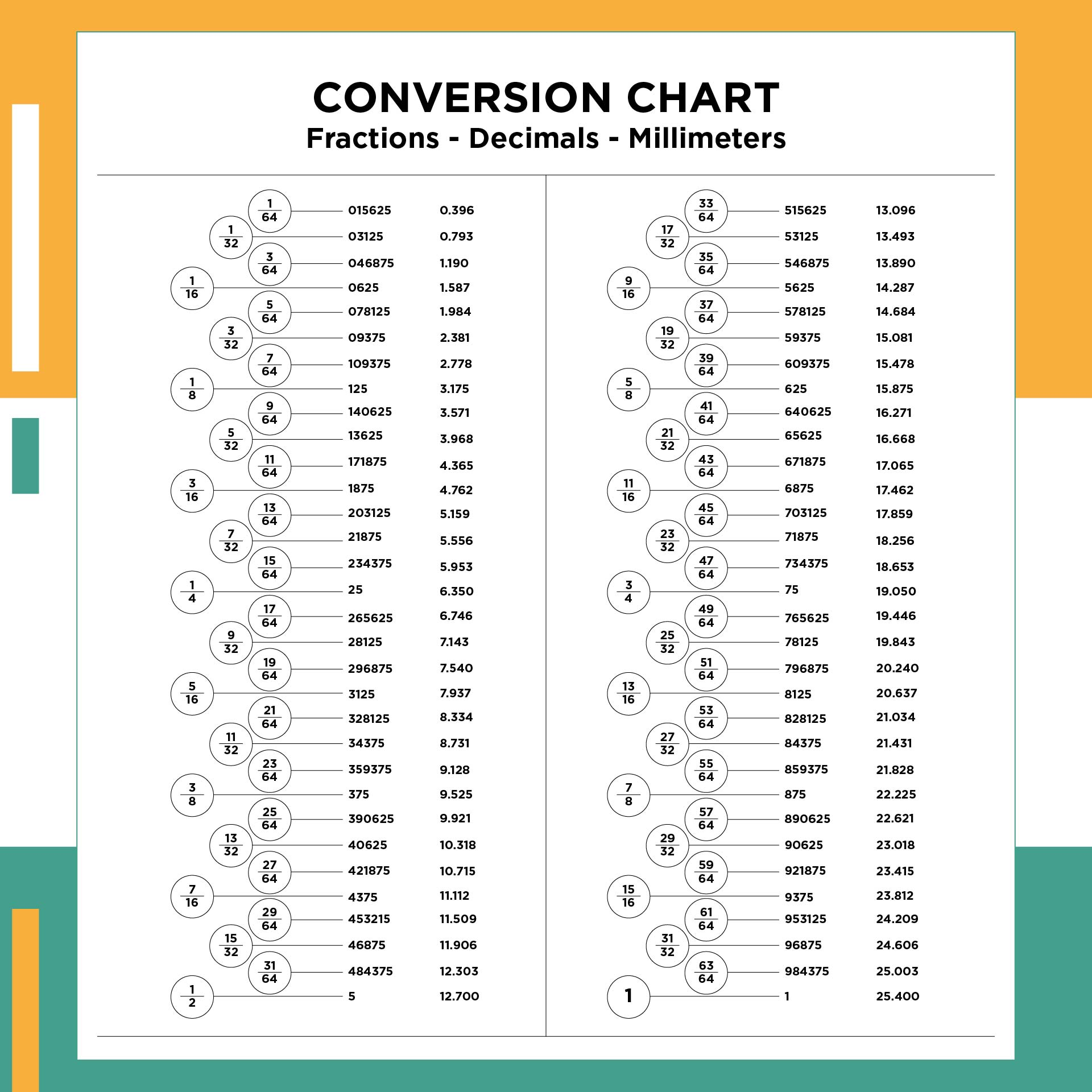We also have more printable chart you may like:
100 Chart Printable
Equivalent Fractions Chart Printable
School Bus Seating Chart Printable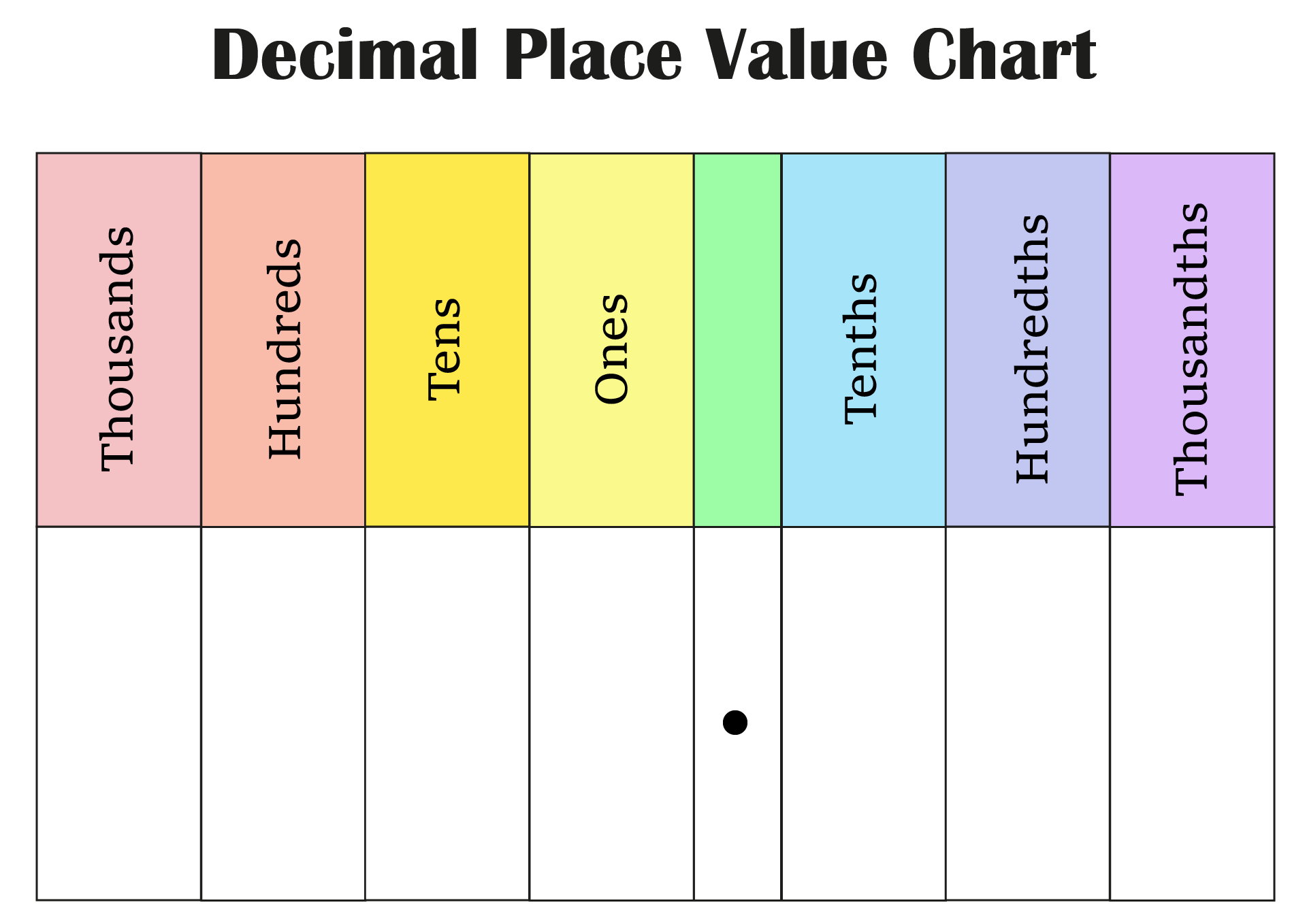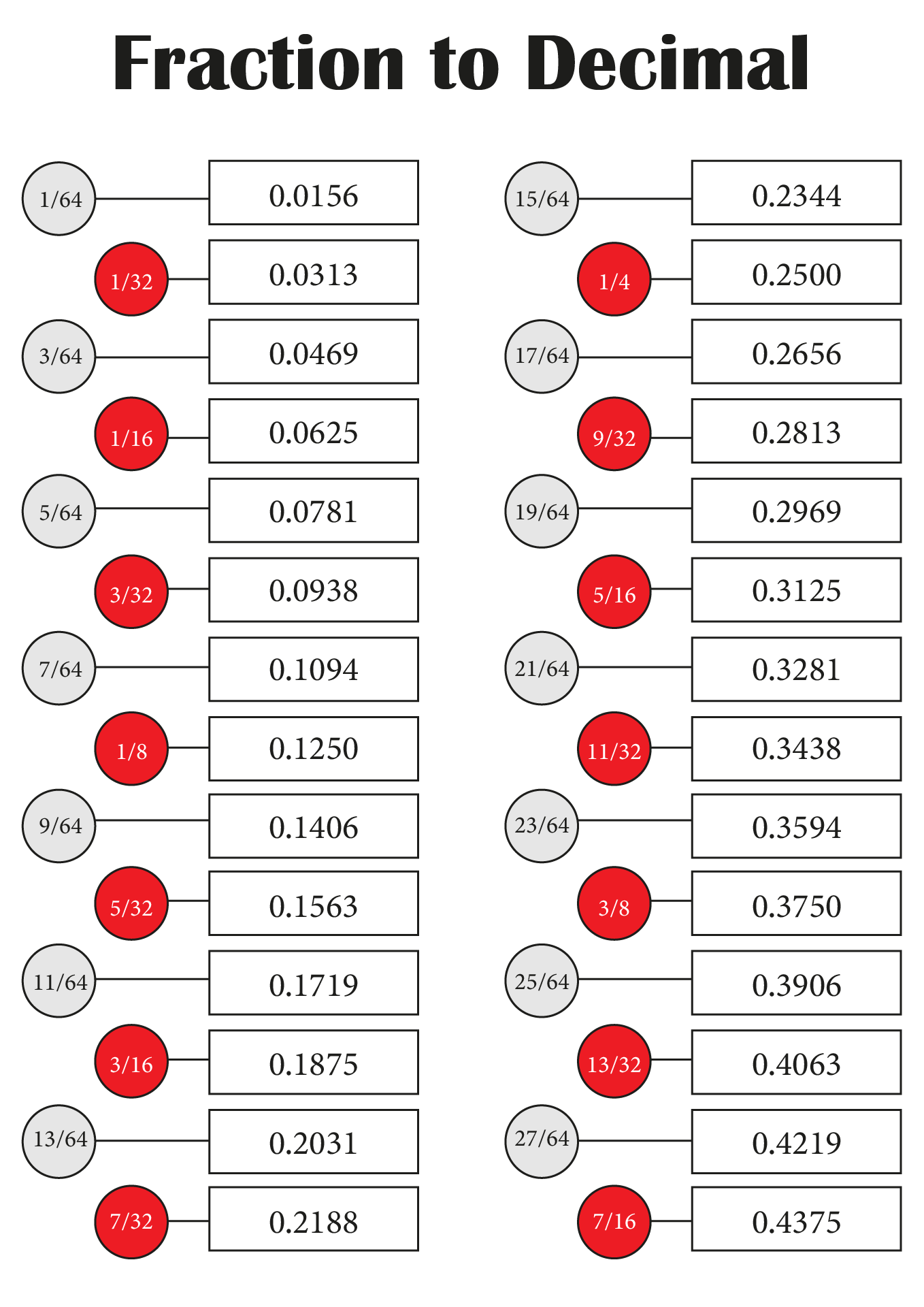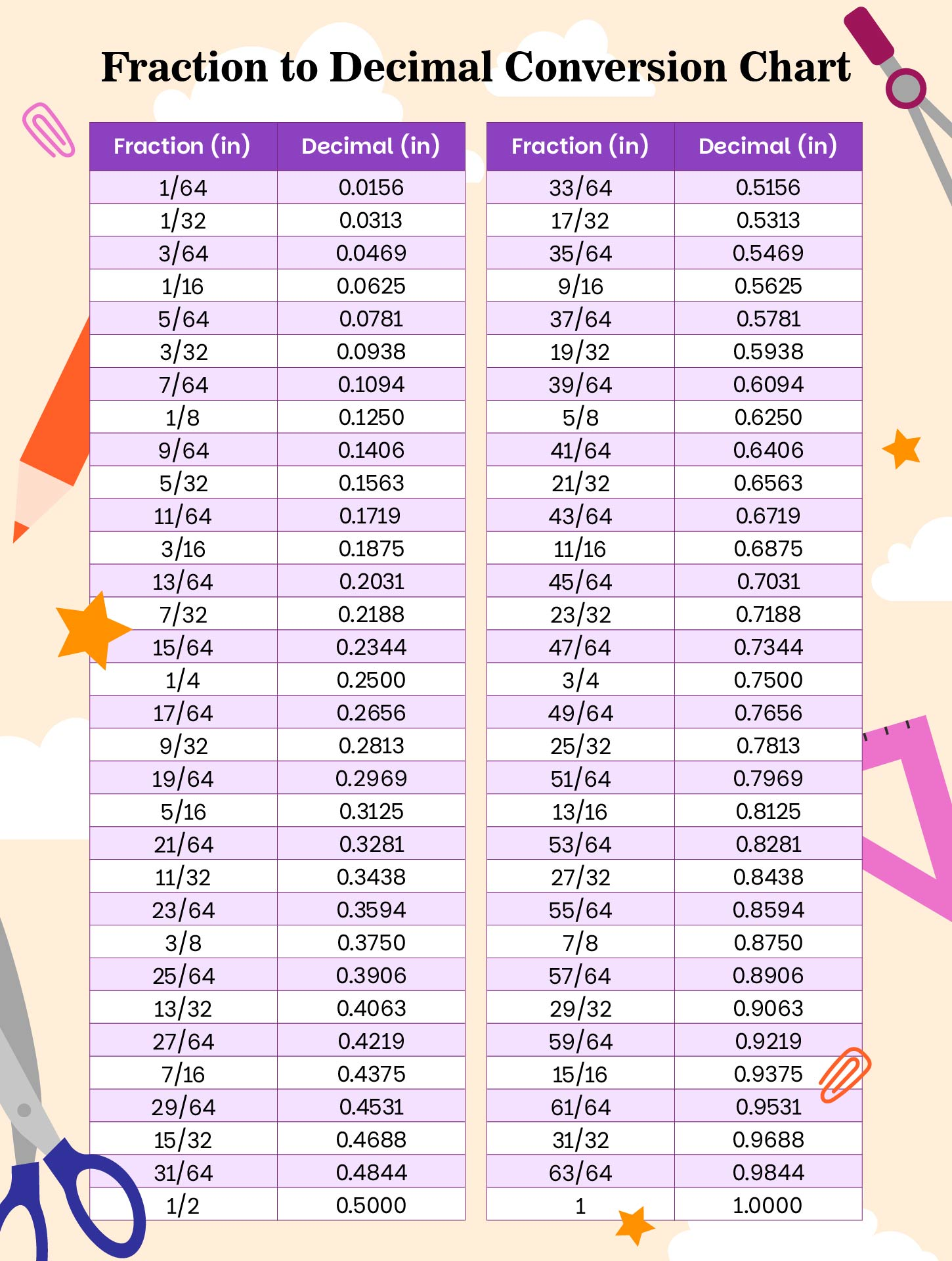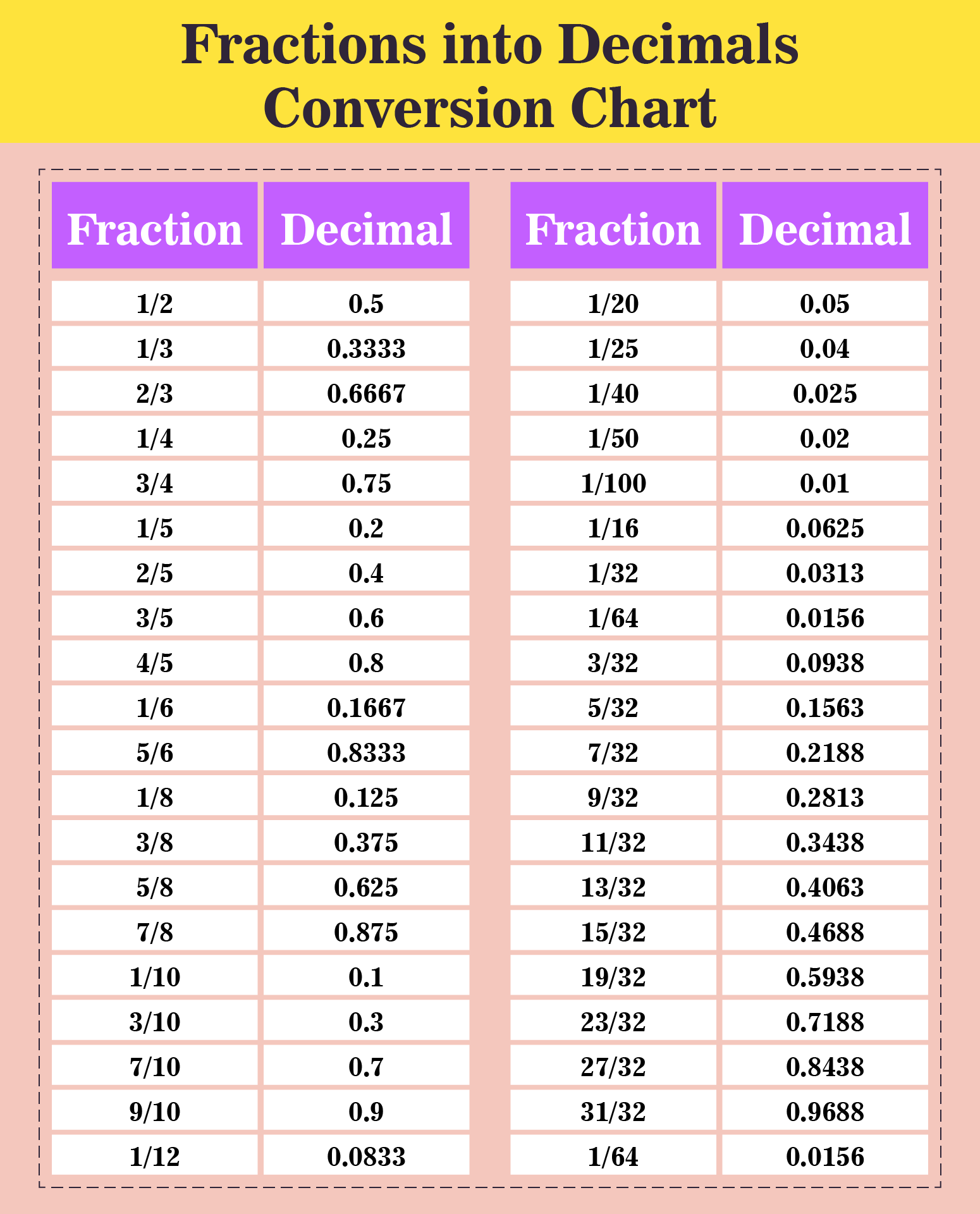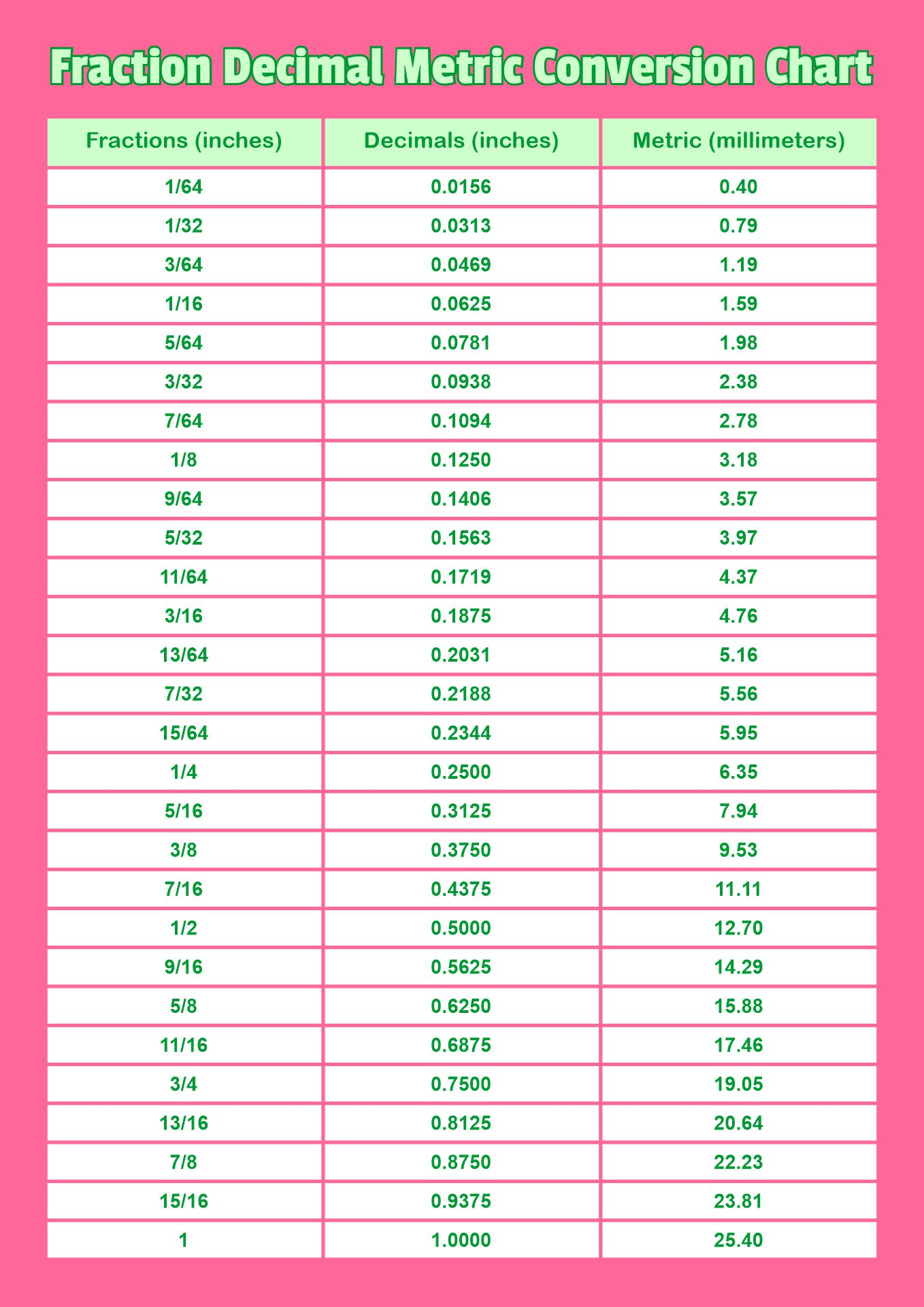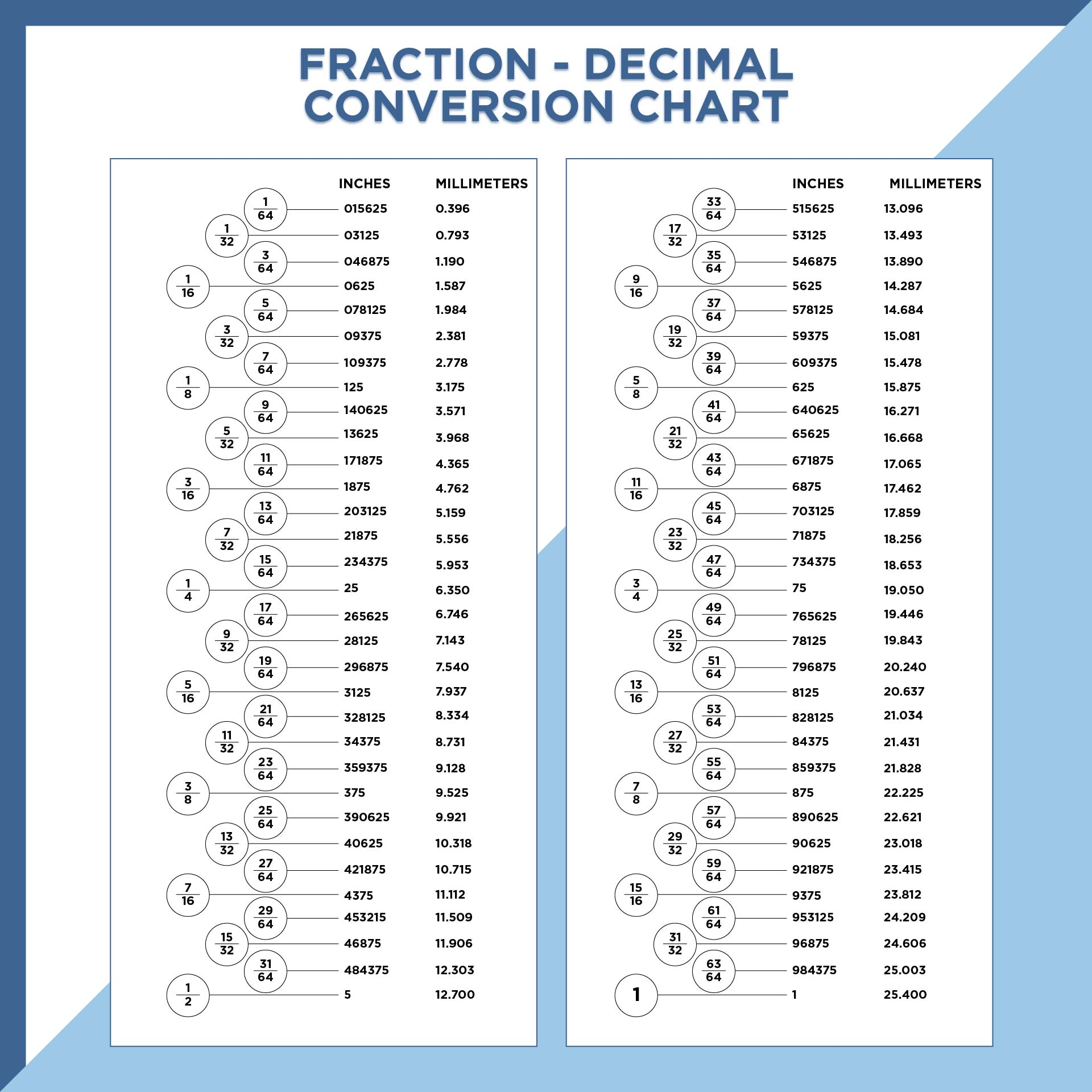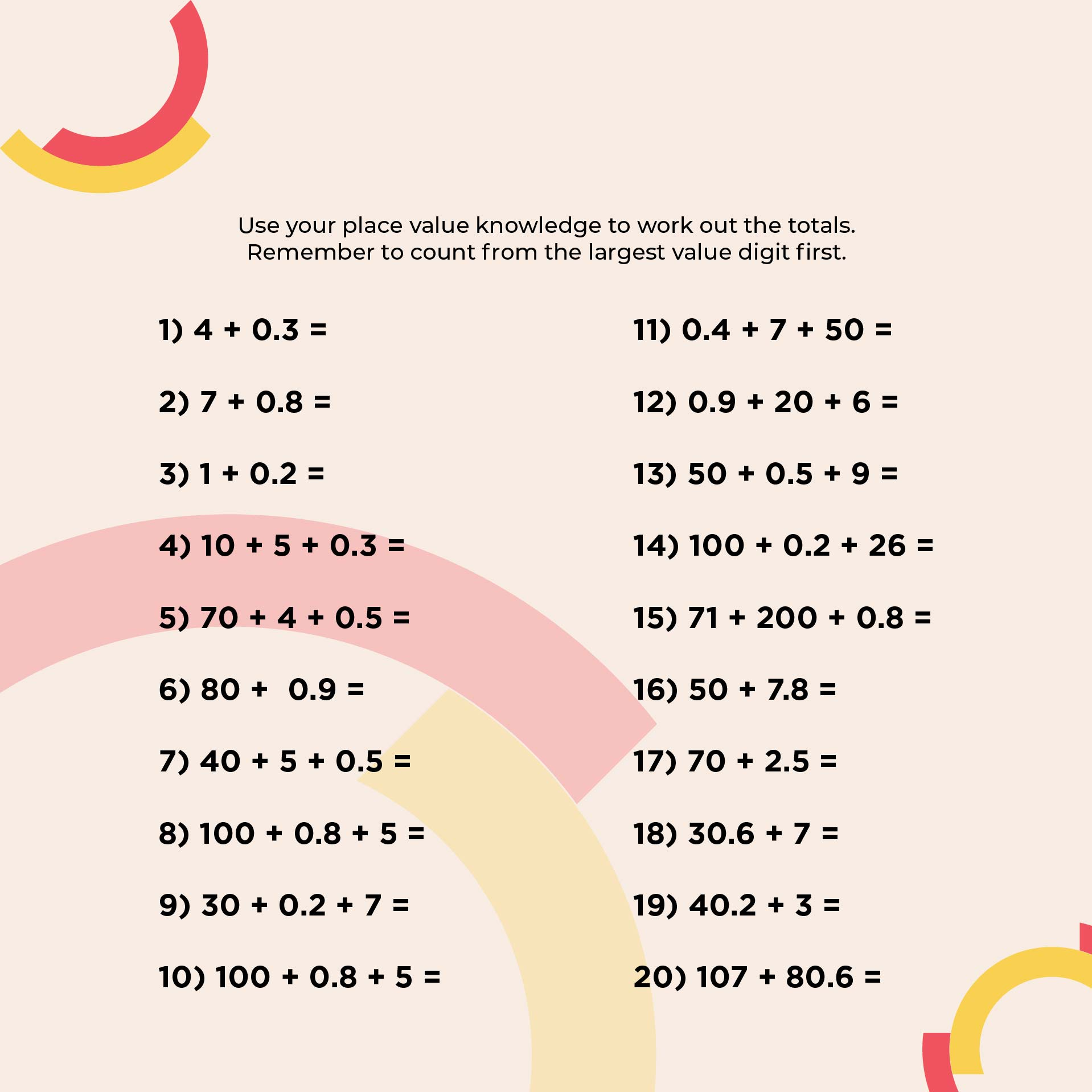## How to Teach Decimals to Kids?

To explain decimals to children, you must introduce them to the basic concept of numbers. The reason is, that decimal is a simple number but has a complicated concept. So, it is important to explain it step by step so children can easily understand it.

It's a good idea to start explaining the concept of decimal numbers to children using integer place values. This is because decimal numbers consist of place values such as hundreds, tens, and units. By explaining the concept of place value, it will be easy for children to understand the concept of decimal numbers.

The way to introduce the basic concept is to write an integer. Then, explain to the children that every number has a place value. You must show the hundreds, tens, and one's values correctly. You can use worksheets or templates to help explain the concept to children.

Explain that decimals are related to fractions. So, you need to explain how to convert fractional numbers to decimal numbers. Then, introduce them by placing a decimal point (comma) in the number. Explain the meaning of the use of the decimal point and when they should use it.

Still related to the use of the decimal point, you need to explain that the decimal point must always be placed to the left of the number. So, the right of the decimal point is a number.

Meanwhile, also explains how to convert fractions into decimals. The trick is to use division. Write down some simple fractions like ½. Then, show them how to convert the fraction to a decimal by dividing 1 by 2. The result is 0.5 which is the decimal form.

If they already understand it, you can teach them how to count decimal numbers. Use simple decimal numbers first. If they are good at it, use more complicated numbers.

To understand the use of decimal numbers and how to write them, children also need to know how to read those numbers. Write some decimal numbers on paper and teach the children how to read the numbers. An example is 0.5 which reads "zero point five."